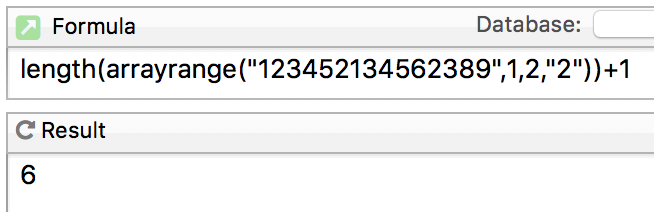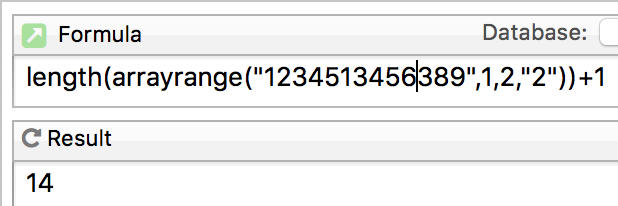# Finding a second instance of a character in a string

I must be searching using the wrong keys or maybe the function that I am looking for does not exist. I need to be able to find the second instance of a specific character in a string. Yes, it is a strange request.You might try using the arrayrange( function, with your character as the separator. Take the length of the first two items and add one, to get the location of the second separator.

This might not be elegant, but to search for the second occurrence of “2” in “123412341234” you could use

``````string="123412341234"
subset=regexreplace(string,"^([^2]+2[^2]+)2.*","\$1")
``````

to find the subset of the original string which precedes the second occurrence of the character, and therefore the index of that second occurrence into the original string is `length(subset)+1` (in this case, 6). If `(length(subset)+1) > length(string)` that indicates no second occurrence has been found.

Of course, to adapt that to search for the second occurrence of an arbitrary character you would have to build the regex search string using `regexliteral(`, because the example I gave would fall down if you tried to find the second occurrence of “.”, for instance.

My solution is using the characterfilter( function to step through the string characters and grab the sequence number of any searchItem found. The array( function gets the second occurrence’s position in the string. Here myString contains the text to be searched and searchItem is the character we are searching for. I have used a chr(1) as a temoporary separator since it is unlikely to used in the original string.

``````array(characterfilter(myString, {?(import()=searchItem,seq()+chr(1),"")}),2,chr(1))
``````

If you leave off the array( function and replace the chr(1) separators with cr() separators you will have a carriage return separated list of the positions of all the searched for characters.

A little late for this question but when you say, “Find the second instance”, my mind asks, “Find the location of the second instance or determine if a second instance exists?” And “Is it guaranteed that there will be a second instance?” Those details would determine a solution path.

I may be wrong but I think, at first glance, that Dave’s solution needs to add 2 rather than one.

And, in Gary’s solution, if the data is defined as:

``````let searchItem = "2"
let myString = "123452134562389"
let nCount = 2
``````

and using “;” as the separator, the result of the `characterfilter(` function would be:

``````2;6;12;
``````

and the expression,

``````array(theResult,nCount,";")
``````

would return the value 6 (the second occurrence), so you have a general expression for the nth position of the search string, with an empty result if there was no such occurrence. You would need to ensure that the chosen separator did not occur in the main string.

James didn’t make it clear whether he just wanted to know the location or if it was to be processed by subsequent code. If the former, there’s no need for the `array(` function, just displaying the result of the `characterfilter(` function would do the job - he could see how many occurrences there were, whether there was indeed a second (or other) occurrence and what its location value was.

One was the correct number.In the formula above, the arrayrange( function returns the string 12345. Then the length function returns 5, and adding 1 gives you 6.

What would it return if there were only one “2”? If arrayrange returns zero (no second “2”), then it looks like “1” is given. Now logically that’s a flag - how can the second occurrence be in the first position - but subsequent code, not considering the significance of 1, might take that number and run with it. Starting conditions are important. Will there always be two or more occurrences of the desired number/character?

If there was no second 2, arrayrange( would return the entire string.The original question seemed to presuppose a second instance of the character, but if that were in doubt, you could test for an arraysize( greater than or equal 3.